Test: Decimals- 2

# Test: Decimals- 2

Test Description

## 10 Questions MCQ Test Mathematics for Class 5 | Test: Decimals- 2

Test: Decimals- 2 for Class 5 2023 is part of Mathematics for Class 5 preparation. The Test: Decimals- 2 questions and answers have been prepared according to the Class 5 exam syllabus.The Test: Decimals- 2 MCQs are made for Class 5 2023 Exam. Find important definitions, questions, notes, meanings, examples, exercises, MCQs and online tests for Test: Decimals- 2 below.
Solutions of Test: Decimals- 2 questions in English are available as part of our Mathematics for Class 5 for Class 5 & Test: Decimals- 2 solutions in Hindi for Mathematics for Class 5 course. Download more important topics, notes, lectures and mock test series for Class 5 Exam by signing up for free. Attempt Test: Decimals- 2 | 10 questions in 15 minutes | Mock test for Class 5 preparation | Free important questions MCQ to study Mathematics for Class 5 for Class 5 Exam | Download free PDF with solutions
 1 Crore+ students have signed up on EduRev. Have you?
Test: Decimals- 2 - Question 1

### If 58 out of 100 students in a school are boys, then write a decimal for the part of school that consists of boys.

Detailed Solution for Test: Decimals- 2 - Question 1

A fraction and a decimal for the part of the school that consists of boys are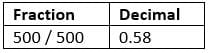Test: Decimals- 2 - Question 2

### 929.5 ÷ 100 is

Detailed Solution for Test: Decimals- 2 - Question 2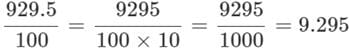Test: Decimals- 2 - Question 3

### 2.1143 × 10 is

Detailed Solution for Test: Decimals- 2 - Question 3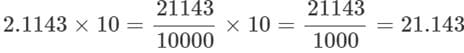Test: Decimals- 2 - Question 4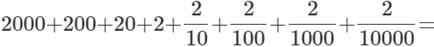Detailed Solution for Test: Decimals- 2 - Question 4

2222 + .2 + .02 + .002 + .0002 = 2222.2222.

Test: Decimals- 2 - Question 5

500.5 × ____ = 5005

Detailed Solution for Test: Decimals- 2 - Question 5

Required number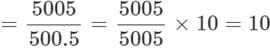Test: Decimals- 2 - Question 6

727.111 ÷ ______ = 7.27111

Detailed Solution for Test: Decimals- 2 - Question 6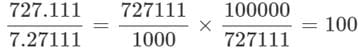Test: Decimals- 2 - Question 7

A baker buys 5 kg of flour and 3.5 kg of sugar for the cake and uses 2.25 kg of flour and .75 kg of sugar in the cake. What is the amount of flour and sugar left with him.

Detailed Solution for Test: Decimals- 2 - Question 7

Amount of flour left = 5.00 - 2.25 = 2.75.
Amount of sugar left = 3.50 - 1.75 = 1.75.

Test: Decimals- 2 - Question 8

The rainfall in a city an first 5 days of a month was 1.27 cm, 3.25 cm, 2.79 cm, 2.57 cm and 1.37 cm. How much did it rain altogether?

Detailed Solution for Test: Decimals- 2 - Question 8

(1.27 + 3.25 + 2.79 + 2.57 + 1.37) cm = 11.25 cm rain.

Test: Decimals- 2 - Question 9

A tailor takes 2.5 m of cloth for making a curtain. He received an order of making 25 curtains from Mrs. Radha puri. How much cloth will he require to fulfill the order?

Detailed Solution for Test: Decimals- 2 - Question 9

Required cloth = (2.5 × 25)m = 62.50 m.

Test: Decimals- 2 - Question 10

Rewrite the following in the decimal form of the given unit 790 m into km.

Detailed Solution for Test: Decimals- 2 - Question 10

1m = 1000 km
∴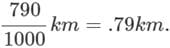## Mathematics for Class 5

12 videos|55 docs|35 tests
 Use Code STAYHOME200 and get INR 200 additional OFF Use Coupon Code
Information about Test: Decimals- 2 Page
In this test you can find the Exam questions for Test: Decimals- 2 solved & explained in the simplest way possible. Besides giving Questions and answers for Test: Decimals- 2, EduRev gives you an ample number of Online tests for practice

## Mathematics for Class 5

12 videos|55 docs|35 tests